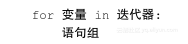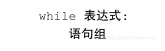《从问题到程序：用Python学编程和计算》——2.8　重复计算和循环

+关注继续查看

2.8.1　重复计算

• 求1到10的整数之和；
• 求2到30的偶数之乘积；
• 求1 – 1/3 + 1/5 – 1/7 + 1/9 …– 1/19，等等。

• 需要做一系列重复性的计算；
• 计算中需要做的操作有规律，可以说清楚。

s = 1 + 2 + 3 + 4 + 5 + 6 + 7 + 8 + 9 + 10
print("The sum is", s)

s = 0
s = s + 1
s = s + 2
s = s + 3
s = s + 4
s = s + 5
s = s + 6
s = s + 7
s = s + 8
s = s + 9
s = s + 10
print("The sum is", s)

Python语言里有两种循环语句：for语句用于描述比较简单比较规范的循环；while语句用于描述一般的复杂循环。它们都控制一个语句组的重复执行。

2.8.2　for语句和重复计算

for语句用一个循环控制器（在Python里称为迭代器）描述其成分语句组的重复执行方式。其基本语法形式是：for和in都是关键字，语句中包含了三个成分，最关键的是迭代器。由关键字for开始的行称为循环的头部，语句组称为循环体。与if语句的情况类似，这里语句组中的语句也是下一层的成分，同样需要退格，组中各语句必须相互对齐。

s = 0
for x in range(100):
s = s + x

• range(n)　得到的迭代器表示序列0, 1, 2, ……, n-1。因此range(100) 表示序列0、1、2、……、99。
• range(m, n)　得到的迭代器表示序列m, m+1, m+2, ……, n-1。例如range(10, 16)表示序列10、11、12、13、14、15。
• range(m, n, d)　得到的迭代器表示等差序列m, m+d, m+2d, ……，按步进值d递增（如果d为负就是递减），直至那个最接近但不包括n的等差值。例如，range(10, 16, 2) 表示的序列是10、12、14，而range(15, 4, -3) 表示的序列是15、12、9、6。

a = int(input("Start from: "))
b = int(input("End at: "))
c = int(input("Step: "))

s = 0
for n in range(a, b+1, c):
s = s + n

print("The sum is", s)

F = C ×9/5＋32

begin = int(input("Start from: "))
end = int(input("End at: "))
step = int(input("Step: "))

for x in range(begin, end, step):
print(x, "->", x * 9 / 5 + 32)

0 -> 32.0
10 -> 50.0
20 -> 68.0
30 -> 86.0
40 -> 104.0

n! = 1×2×…×n

0的阶乘定义为1。完成这个计算需要乘起一系列整数，直至某个在写程序时不能确定的n。这种工作必须用循环处理。在重复计算中需记录不断增长的部分乘积，使用一个变量。

n = int(input("Factorial for: "))

prod = 1
for i in range(2, n + 1):
prod = prod * i

print("The factorial of", n, "is", prod)

for i in range(3):
n = int(input("Factorial for: "))

prod = 1
for j in range(2, n+1):
prod = prod * j

print("The factorial of", n, "is", prod)

2.8.3　while语句和迭代n = int(input("Factorial for: "))

prod = 1
i = 2
while i <= n:
prod = prod * i
i = i + 1

print("The factorial of", n, "is", prod)

print("This is a factorial calculator. -1 to stop.")
n = int(input("Factorial for: "))

while n >= 0:
prod = 1
for i in range(2, n+1):
prod = prod * i

print("The factorial of", n, "is", prod)

n = int(input("Factorial for: "))

print("Bye!")

>>>
This is a factorial calculator. -1 to stop.
Factorial for: 10
The factorial of 10 is 3628800
Factorial for: 5
The factorial of 5 is 120
Factorial for: -1
Bye!

0.假设要求实数x的平方根，任取y为某个实数值
1.如果y×y＝x，计算结束，y就是x的平方根
2.令z＝(y＋x/y)/2
3.令y的新值为z，转回步骤1

x = float(input("Square root for: "))

guess = 1.0

while guess * guess != x:
guess = (guess + x/guess)/2

print(guess)

x = float(input("Square root for: "))

guess = 1.0

while guess * guess != x:
guess = (guess + x/guess)/2
print(guess, guess * guess)

print(guess)

x = float(input("Square root for: "))

guess = 1.0

while abs(guess * guess - x) > 1e-8:
guess = (guess + x/guess)/2

print(guess)

x = float(input("Square root for: "))

guess = 1.0
n = 0

while abs(guess * guess - x) > 1e-8:
guess = (guess + x/guess)/2
n = n + 1
print(n, guess)

print(guess)

Square root for: 2.0
1 1.5
2 1.4166666666666665
3 1.4142156862745097
4 1.4142135623746899
1.4142135623746899

Python的一个优点是很容易做各种计算试验，本质上是利用计算机可编程的优点。但Python语言的设计使人在做这些事情时非常方便。读者可以自己考虑一些有趣的计算问题，做些试验，观察分析计算中的情况和问题。

2.8.4　循环控制

for语句和while语句都是通过头部控制循环的进行，一旦执行进入循环体，就会完整地执行一遍其中的语句，然后再重复。实际中也存在一些情况，其中在一定条件下应该只执行循环体的一部分，然后就退出循环，或者立刻转去做循环的下一次迭代。为了满足这类需要，Python提供了两个特殊的循环控制语句。

print("This is a factorial calculator. -1 to stop.")

while True:
n = int(input("Factorial for: "))
if n < 0:
break

prod = 1
for i in range(2, n+1):
prod = prod * i

print("The factorial of", n, "is", prod)

print("Bye!").NET Core是一个开源通用的开发框架，支持跨平台, 阿里云函数计算推出了 dotnetcore2.1 runtime， 使用 C# 编写 serverless 函数， 除了很好地支持通常意义上的函数外， 还可以基于函数计算开发 asp.
4547 0Python编程语言学习：仅需一行代码构造特殊列表之重复元素列表、等差数字列表等之详细攻略
Python编程语言学习：仅需一行代码构造特殊列表之重复元素列表、等差数字列表等之详细攻略
17 01243 0【转】C# 计算程序运行时间
1 //计算程序运行时间(.net1.1 于.net2.0的区别)在.net2.0中提供了Stopwatch类，简单例子  2 using System.Diagnostics;  3  4 private Stopwatch stw = new Stopwatch();  5  6 pri...
600 0《从问题到程序：用Python学编程和计算》——2.11　补充材料
1748 0《好学的C++程序设计》——1.1 计算机怎样计数
1438 0《并行计算的编程模型》一3.7.1　选择集合参与者

846 0EasyUI中那些不容易被发现的坑——EasyUI重复请求2次的问题
909 0《从问题到程序：用Python学编程和计算》——练习
5144 0《Excel 职场手册：260招菜鸟变达人》一第 16 招　利用数据验证防止重复录入相同的内容
1268 0
10059

0

+ 订阅

《2021云上架构与运维峰会演讲合集》

《零基础CSS入门教程》

《零基础HTML入门教程》